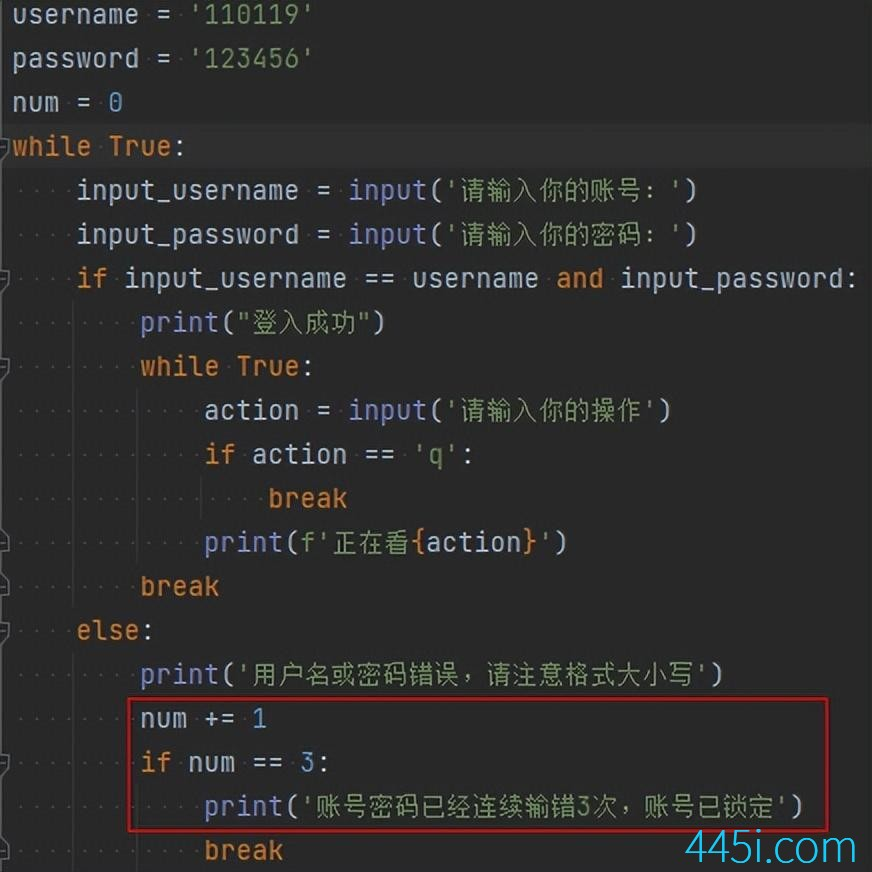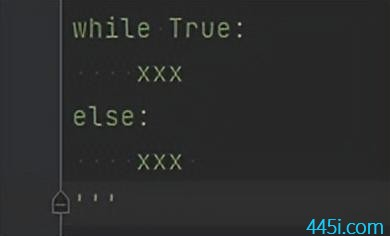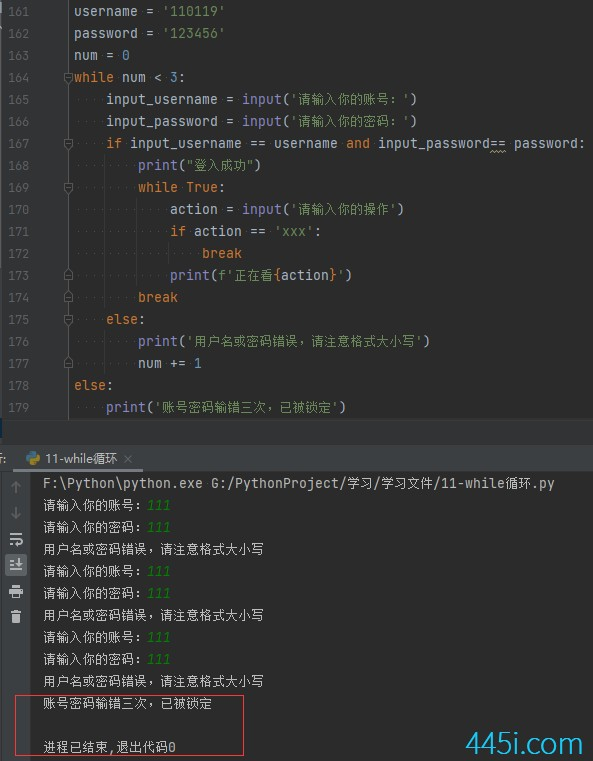# Python while与else

``````username = '110119'
while True:
print("登入成功")
while True:
action = input('请输入你的操作')
if action == 'xxx':
break
print(f'正在看{action}')
break
else:
print('用户名或密码错误，请注意格式大小写')``````

``````username = '110119'
num = 0
while True:``````username = '110119'
num = 0
while True:
print("登入成功")
while True:
action = input('请输入你的操作')
if action == 'q':
break
print(f'正在看{action}')
break
else:
print('用户名或密码错误，请注意格式大小写')
num += 1
if num == 3:
print('账号密码已经连续输错3次，账号已锁定')
break``````while加else，用法很简单，就直接加在while循环整个代码块后面就行了。``````username = '110119'
num = 0
while num < 3:
print("登入成功")
while True:
action = input('请输入你的操作')
if action == 'xxx':
break
print(f'正在看{action}')
break
else:
print('用户名或密码错误，请注意格式大小写')
num += 1
else:
print('账号密码输错三次，已被锁定')``````### 觉得文章有用就打赏一下文章作者

#### 支付宝扫一扫打赏#### 微信扫一扫打赏